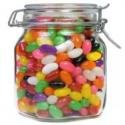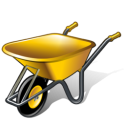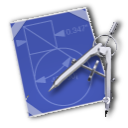# Category: Area# How Many Cubic Inches In A Jar Or Cylinder Calculator

To calculate the cubic inches inside a jar or other cylinder shaped object, you will need to know the diameter and height of the jar then apply the following formula:

Let’s say our jar is 8 inches … Continue Reading →# How Many Jelly Beans In A Jar Calculator

Trying to figure out how many jelly beans there are in a mason jar or gallon jug? Here’s our method of getting the number, but you can jump to the bottom and just use the calculator too.

One way … Continue Reading →# Calculate The Area Of A Circle

To calculate the area of a circle, use the following formula:

Let’s say our circle is 8 inches in diameter. Half of the circle’s diameter equals it’s radius (8 / 2 = 4). Square the radius (4 * 4 = … Continue Reading →# Topsoil Cubic Feet Yards And Bags Calculator

The Topsoil Calculator will help you figure out how much topsoil or potting soil to buy, with a breakdown in terms of bags or yards. It will convert your measurements into cubic feet and yards so you … Continue Reading →# Calculate The Area Of A Trapezium

To calculate the area of a trapezium, which is essentially a rectangle with triangles on either side:

add together the top and bottom (top + bottom)
multiply the result with the height ((top + bot … Continue Reading →# Calculate The Area Of A Triangle

To calculate the area of a triangle:

multiply the base with the height (base * height)
divide the result in half ((base * height) / 2) … Continue Reading →# Calculate The Area Of A Parallelogram

To calculate the area of a Parallelogram, use the following formula:

multiply the width with the height (width * height) … Continue Reading →# Calculate The Area Of A Rectangle

To calculate the area of a Rectangle, use the following formula:

multiply the width with the height (width * height) … Continue Reading →# Circumference Calculator

Get the circumference of a circle by entering its radius. … Continue Reading →